# Differential Equations Class 12 NCERT Solutions

NCERT Solutions Class 12 Mathematics Chapter 9 Differential Equations Download In Pdf

## In this pdf file you can see answers of following Questions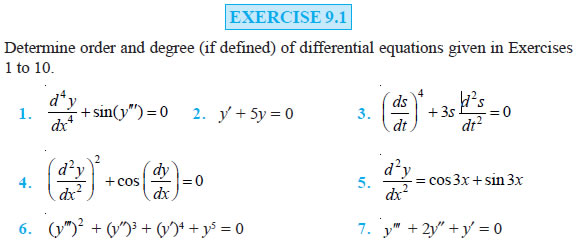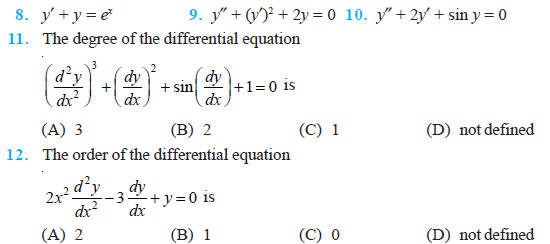### EXERCISE 9.2

In each of the Exercises 1 to 10 verify that the given functions (explicit or implicit) is a solution of the corresponding differential equation:

Question 1. y = ex + 1 : y″ – y′ = 0

Question 2. y = x2 + 2x + C : y′ – 2x – 2 = 0

Question 3. y = cos x + C : y′ + sin x = 0

Question 4. y = 1+ x2 : y′ = 1 2 xy + x

Question 5. y = Ax : xy′ = y (x ≠ 0)

Question 6. y = x sin x : xy′ = y + x x2 − y2 (x ≠ 0 and x > y or x < – y)

Question 7. xy = log y + C : y′ = 2 1 y − xy (xy ≠ 1)

Question 8. y – cos y = x : (y sin y + cos y + x) y′ = y

Question 9. x + y = tan–1y : y2 y′ + y2 + 1 = 0

Question 10. y = a2 − x2 x ∈ (–a, a) : x + y dy dx = 0 (y ≠ 0)

Question 11. The number of arbitrary constants in the general solution of a differential equation of fourth order are:
(A) 0
(B) 2
(C) 3
(D) 4

Question 12. The number of arbitrary constants in the particular solution of a differential equation of third order are:
(A) 3
(B) 2
(C) 1
(D) 0

### EXERCISE 9.3

In each of the Exercises 1 to 5, form a differential equation representing the given family of curves by eliminating arbitrary constants a and b.

Question 1. x y 1 a b + =

Question 2. y2 = a (b2 – x2)

Question 3. y = a e3x + b e– 2x

Question 4. y = e2x (a + bx)

Question 5. y = ex (a cos x + b sin x)

Question 6. Form the differential equation of the family of circles touching the y-axis at origin.

Question 7. Form the differential equation of the family of parabolas having vertex at origin and axis along positive y-axis.

Question 8. Form the differential equation of the family of ellipses having foci on y-axis and centre at origin.

Question 9. Form the differential equation of the family of hyperbolas having foci on x-axis and centre at origin.

Question 10. Form the differential equation of the family of circles having centre on y-axis and radius 3 units.

Question 11. Which of the following differential equations has y = c1 ex + c2 e–x as the general solution?
(A) 2 2 d y y 0 dx + =
(B) 2 2 d y y 0 dx − =
(C) 2 2 d y 1 0 dx + =
(D) 2 2 d y 1 0 dx − =

Question 12. Which of the following differential equations has y = x as one of its particular solution?
(A) 2 2 2 d y x dy xy x dx dx − + =
(B) 2 2 d y x dy xy x dx dx + + =
(C) 2 2 2 d y x dy xy 0 dx dx − + =
(D) 2 2 d y x dy xy 0 dx dx |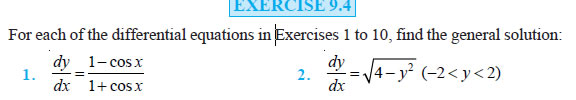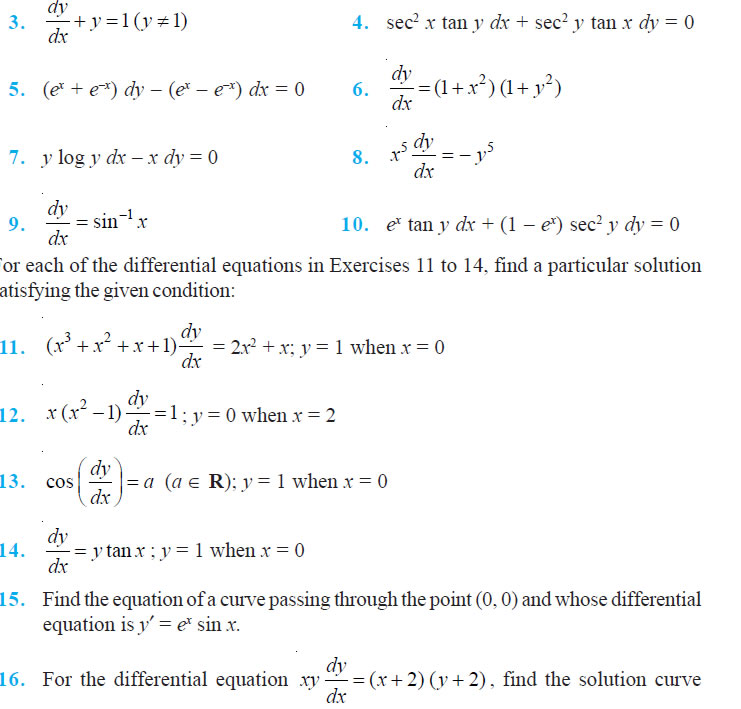Question 17. Find the equation of a curve passing through the point (0, –2) given that at any point (x, y) on the curve, the product of the slope of its tangent and y coordinate of the point is equal to the x coordinate of the point.

Question 18. At any point (x, y) of a curve, the slope of the tangent is twice the slope of the line segment joining the point of contact to the point (– 4, –3). Find the equation of the curve given that it passes through (–2, 1).

Question 19. The volume of spherical balloon being inflated changes at a constant rate. If initially its radius is 3 units and after 3 seconds it is 6 units. Find the radius of balloon after t seconds.

Question 20. In a bank, principal increases continuously at the rate of r% per year. Find the value of r if Rs 100 double itself in 10 years (loge2 = 0.6931).

Question 21. In a bank, principal increases continuously at the rate of 5% per year. An amount of Rs 1000 is deposited with this bank, how much will it worth after 10 years (e0.5 = 1.648).

Question 22. In a culture, the bacteria count is 1,00,000. The number is increased by 10% in 2 hours. In how many hours will the count reach 2,00,000, if the rate of growth of bacteria is proportional to the number present?

Question 23. The general solution of the differential equation dy ex y dx = + is (A) ex + e–y = C (B) ex + ey = C (C) e–x + ey = C (D) e–x + e–y = C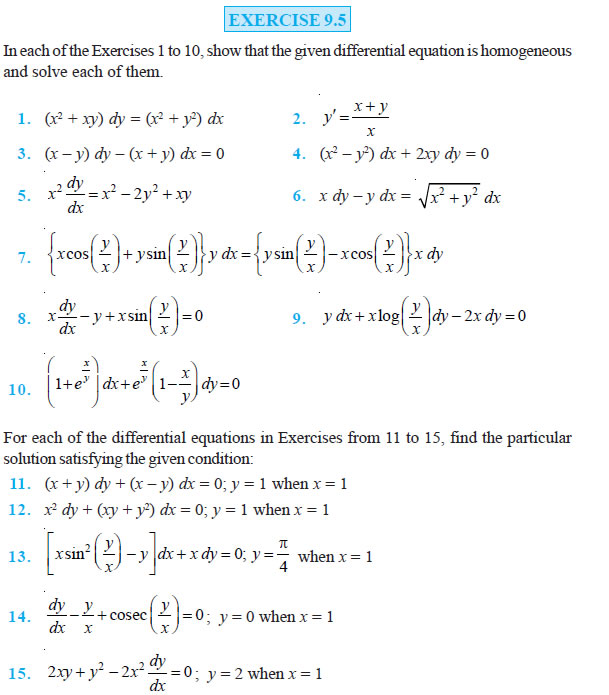Question 16. A homogeneous differential equation of the from dx h x dy y = can be solved by making the substitution.
(A) y = vx
(B) v = yx
(C) x = vy
(D) x = v

Question 17. Which of the following is a homogeneous differential equation?
(A) (4x + 6y + 5) dy – (3y + 2x + 4) dx = 0
(B) (xy) dx – (x3 + y3) dy = 0
(C) (x3 + 2y2) dx + 2xy dy = 0
(D) y2 dx + (x2 – xy – y2) dy = 0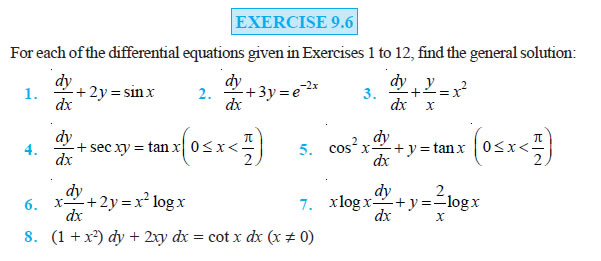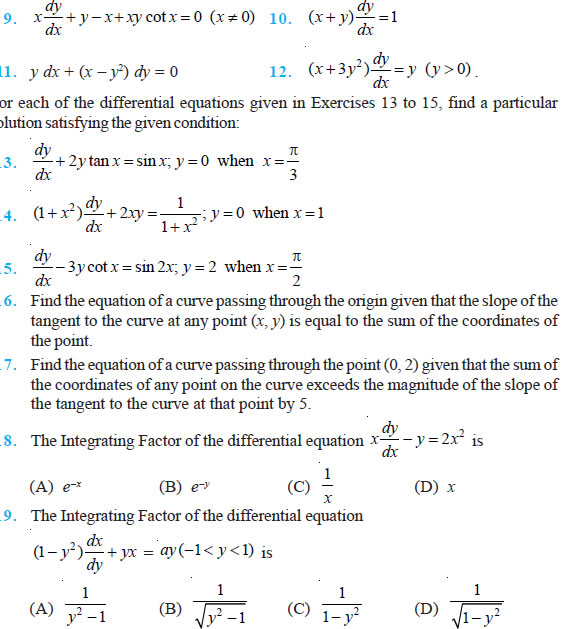### Miscellaneous Exercise on Chapter 9

Question 1. For each of the differential equations given below, indicate its order and degree (if defined).
(i) 2 2 2 d y 5x dy 6y log x dx dx +
(ii) 3 2 dy 4 dy 7 y sin x dx dx
(iii) 4 3 4 3 d y sin d y 0 dx dx

Question 2. For each of the exercises given below, verify that the given function (implicit or explicit) is a solution of the corresponding differential equation.
(i) y = a ex + b e–x + x2 : 2 2 2 x d y 2 dy xy x 2 0 dx dx + − + − =
(ii) y = ex (a cos x + b sin x) : 2 2 d y 2 dy 2y 0 dx dx − + =
(iii) y = x sin 3x : 2 2 d y 9y 6cos3x 0 dx + − =
(iv) x2 = 2y2 log y : (x2 y2 ) dy xy 0 dx + − =

Question 3. Form the differential equation representing the family of curves given by (x – a)2 + 2y2 = a2, where a is an arbitrary constant.

Question 4. Prove that x2 – y2 = c (x2 + y2)2 is the general solution of differential equation (x3 – 3x y2) dx = (y3 – 3x2y) dy, where c is a parameter.

Question 5. Form the differential equation of the family of circles in the first quadrant which touch the coordinate axes.

Question 6. Find the general solution of the differential equation 2 2 1 0 1 dy y dx x − + = − .

Question 7. Show that the general solution of the differential equation 2 2 1 0 1 dy y y dx x x + + + = + + is given by (x + y + 1) = A (1 – x – y – 2xy), where A is parameter.

Question 8. Find the equation of the curve passing through the point 0, 4 whose differential equation is sin x cos y dx + cos x sin y dy = 0.

Question 9. Find the particular solution of the differential equation (1 + e2x) dy + (1 + y2) ex dx = 0, given that y = 1 when x = 0.

Question 10. Solve the differential equation 2 ( 0) x x y e ydx x e y y dy y ≠ .

Question 11. Find a particular solution of the differential equation (x – y) (dx + dy) = dx – dy, given that y = –1, when x = 0. (Hint: put x – y = t)

Question 12. Solve the differential equation 2 1( 0) x e y dxx x x dy.

Question 13. Find a particular solution of the differential equation cot dy y x dx + = 4x cosec x (x ≠ 0), given that y = 0 when 2 x π = .

Question 14. Find a particular solution of the differential equation (x + 1) dy dx = 2 e–y – 1, given that y = 0 when x = 0.

Question 15. The population of a village increases continuously at the rate proportional to the number of its inhabitants present at any time. If the population of the village was 20, 000 in 1999 and 25000 in the year 2004, what will be the population of the village in 2009?

Question 16. The general solution of the differential equation y dx x dy 0 y − = is
(A) xy = C
(B) x = Cy2
(C) y = Cx
(D) y = Cx2

Question 17. The general solution of a differential equation of the type P1 Q1 dx x dy + = is
(A) P1 ( P1 ) Q1 C dy dy y e∫ = ∫ e∫ dy +
(B) P1 ( P1 ) . Q1 C dx dx y e∫ = ∫ e∫ dx +
(C) P1 ( P1 ) Q1 C dy dy x e∫ = ∫ e∫ dy +
(D) P1 ( P1 ) Q1 C dx dx x e∫ = ∫ e∫ dx +

Question 18. The general solution of the differential equation ex dy + (y ex + 2x) dx = 0 is
(A) x ey + x2 = C
(B) x ey + y2 = C
(C) y ex + x2 = C
(D) y ey + x2 = C

### NCERT Books Free Pdf Download for Class 5, 6, 7, 8, 9, 10 , 11, 12 Hindi and English Medium

 Mathematics Biology Psychology Chemistry English Economics Sociology Hindi Business Studies Geography Science Political Science Statistics Physics Accountancy